爬虫学习（10）：python正则表达式大全

18 篇文章 270 订阅

正则表达式模块

Python 有一个名为 的内置包re，可用于处理正则表达式。导入re模块：

import re

Python中的正则表达式

import re

txt = "The rain in Spain"
x = re.search("^The.*Spain$", txt) if x: print("匹配成功!") else: print("匹配失败") 运行：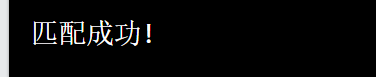当然，你现在看不懂这个例子，既然手把手教学，并不会教大家一步登天。 正则表达式函数 findall() 函数 该findall()函数返回一个包含所有匹配项的列表。 例如：打印所有匹配项的列表 import re txt = "川川菜鸟啊菜鸟啊" x = re.findall("菜鸟", txt) print(x) 运行返回：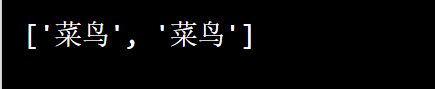该列表按找到的顺序包含匹配项。如果未找到匹配项，则返回一个空列表： import re txt = "菜鸟并不菜" x = re.findall("川川", txt) print(x) if (x): print("匹配成功了哟") else: print("找不到这个呀！") 运行返回：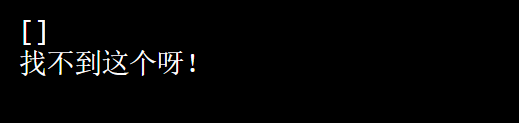search() 函数 该search()函数在字符串中搜索匹配项，如果有匹配项，则返回一个Match 对象。如果有多个匹配项，则只返回匹配项的第一次出现。 例如：搜索字符串中的第一个空白字符： import re txt = "菜鸟 呢" x = re.search("\s", txt) print("第一个空格字符位于位置:", x.start()) 运行结果：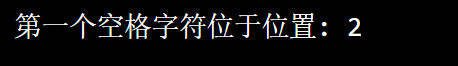如果未找到匹配项，None则返回该值： import re txt = "天上飞的是菜鸟" x = re.search("川川", txt) print(x) 返回：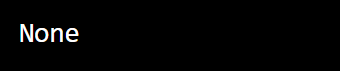split() 函数 该split()函数返回一个列表，其中的字符串在每次匹配时被拆分。 例如：在每个空白字符处拆分 import re txt = "菜鸟 学 python" x = re.split("\s", txt) print(x) 运行返回：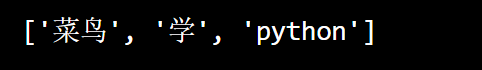您可以通过指定maxsplit 参数来控制出现次数 例如：仅在第一次出现时拆分字符串： import re #Split the string at the first white-space character: txt = "飞起来 菜鸟 们" x = re.split("\s", txt, 1) print(x) 返回：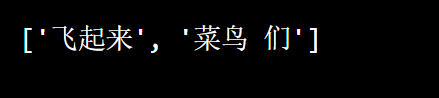sub() 函数 该sub()函数用您选择的文本替换匹配项。 例如：用只替换就 import re txt = "学python就找川川菜鸟" x = re.sub("就", "只", txt) print(x) 运行：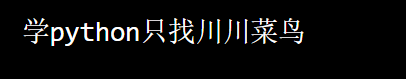您可以通过指定count 参数来控制替换次数 ： 例如替换前 2 次出现： import re txt = "学python就就就川川菜鸟" x = re.sub("就", "只", txt,2) print(x) 返回：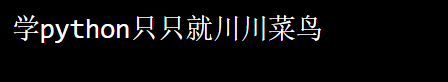元字符 列表符号 [] 用于一组字符 例如：#按字母顺序查找“a”和“m”之间的所有小写字符 import re txt = "apple chuanchuan " #按字母顺序查找“a”和“m”之间的所有小写字符 x = re.findall("[a-m]", txt) print(x) 运行：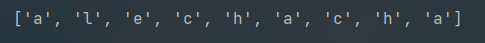转义符 ** 表示特殊序列（也可用于转义特殊字符） 例如匹配所有数字： import re txt = "我今年20岁了" #查找所有数字字符 x = re.findall("\d", txt) print(x) 运行返回：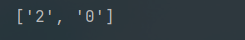任意符号 . 可以任何字符（换行符除外）。 例如：搜索以“he”开头、后跟两个（任意）字符和一个“o”的序列 import re txt = "hello world" #搜索以“he”开头、后跟两个（任意）字符和一个“o”的序列 x = re.findall("he..o", txt) print(x) 运行返回：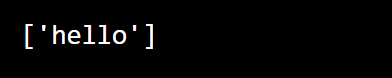开始符 ^符号用于匹配开始。 import re txt = "川川菜鸟 飞起来了" x = re.findall("^川", txt) if x: print("哇，我匹配到了") else: print("哎呀，匹配不了啊") 运行：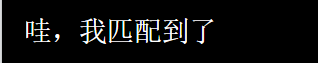结束符$ 符号用于匹配结尾，例如：匹配字符串是否以“world”结尾

import re

txt = "hello world"

#匹配字符串是否以“world”结尾

x = re.findall("world$", txt) if x: print("匹配成功了耶") else: print("匹配不到哦") 运行：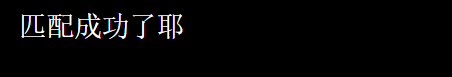星号符 • 星号符用于匹配零次或者多次出现。 import re txt = "天上飞的是菜鸟，学python找川川菜鸟!" #检查字符串是否包含“ai”后跟 0 个或多个“x”字符： x = re.findall("菜鸟*", txt) print(x) if x: print("匹配到了!") else: print("气死了，匹配不到啊") 运行：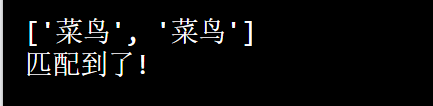加号符 + 用于匹配一次或者多次出现 例如：检查字符串是否包含“菜鸟”后跟 1 个或多个“菜鸟”字符： import re txt = "飞起来了，菜鸟们!" #检查字符串是否包含“菜鸟”后跟 1 个或多个“菜鸟”字符： x = re.findall("菜鸟+", txt) print(x) if x: print("匹配到了!") else: print("烦死了，匹配不到") 运行：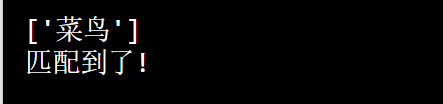集合符号 {} 恰好指定的出现次数 例如：检查字符串是否包含“川”两个 import re txt = "川川菜鸟并不菜!" #检查字符串是否包含“川”两个 x = re.findall("川{2}", txt) print(x) if x: print("匹配到了两次的川") else: print("匹配不到啊，帅哥") 返回：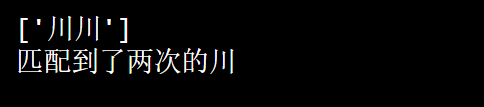或符 | 匹配两者任一 例如：匹配字符串菜鸟或者是我了 import re txt = "菜鸟们学会python了吗？串串也是菜鸟啊!" x = re.findall("菜鸟|是我了", txt) print(x) if x: print("匹配到了哦！") else: print("匹配失败") 运行：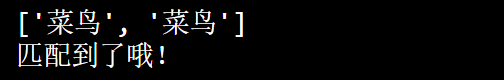特殊序列 指定字符 \A ： 如果指定的字符位于字符串的开头，则返回匹配项。 例如：匹配以菜字符开头的字符 import re txt = "菜鸟在这里" x = re.findall("\A菜", txt) print(x) if x: print("是的匹配到了") else: print("匹配不到") 运行：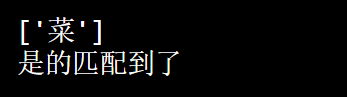指定开头结尾 \b 返回指定字符位于单词开头或结尾的匹配项 （开头的“r”确保字符串被视为原始字符串）。 例如：匹配爱开头 import re txt = "爱你，川川" x = re.findall(r"\b爱", txt) print(x) if x: print("匹配到了") else: print("匹配不到") 运行：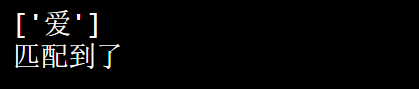又例如：匹配川结尾 import re txt = "爱你，川川" x = re.findall(r"川\b", txt) print(x) if x: print("匹配到了") else: print("匹配不到") 运行：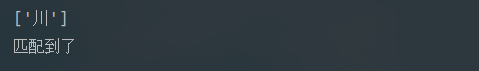匹配中间字符 \B 返回存在指定字符但不在单词开头（或结尾）的匹配项 （开头的“r”确保字符串被视为“原始字符串”） 比如我匹配菜鸟： import re txt = "我是菜鸟我是菜鸟啊" #检查是否存在“ain”，但不是在单词的开头： x = re.findall(r"\菜鸟", txt) print(x) if x: print("匹配到了嘛！!") else: print("匹配不到哇！") 运行：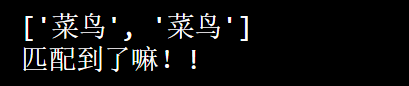但是你匹配结尾就会返回空，比如我匹配鸟： import re txt = "川川菜鸟" #检查是否存在“鸟”，但不是在单词的末尾： x = re.findall(r"鸟\B", txt) print(x) if x: print("匹配到了哦") else: print("找不到") 运行：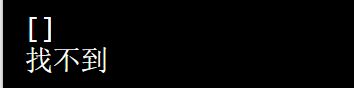匹配数字 \d 返回字符串包含数字（0-9 之间的数字）的匹配项。 例如： import re txt = "我今年20岁了啊" #检查字符串是否包含任何位数（0-9的数字） x = re.findall("\d", txt) print(x) if x: print("哇哇哇，匹配到数字了") else: print("找不到哦") 运行：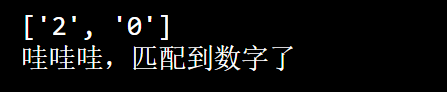匹配非数字 \D 返回字符串不包含数字的匹配项 例如： import re txt = "我今年20岁" #匹配任何非数字符号 x = re.findall("\D", txt) print(x) if x: print("匹配到了，开心!") else: print("匹配不到，生气") 运行：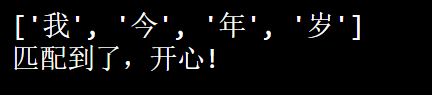空格匹配 \s 返回一个匹配字符串包含空白空间字符的匹配项。 例如： import re txt = "我 是 川 川 菜 鸟" #匹配任何空格字符 x = re.findall("\s", txt) print(x) if x: print("匹配到了") else: print("匹配不到啊") 运行：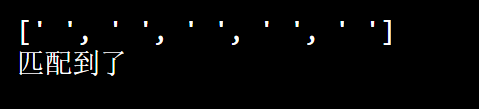匹配非空格 \S 返回字符串不包含空格字符的匹配项 import re txt = "菜鸟是 我 了" #匹配任意非空字符 x = re.findall("\S", txt) print(x) if x: print("匹配到了!") else: print("匹配不到啊") 运行：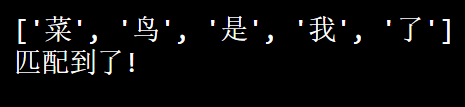匹配任意数字和字母 返回一个匹配，其中字符串包含任何单词字符（从 a 到 Z 的字符，从 0 到 9 的数字，以及下划线 _ 字符） 例如： import re txt = "菜鸟啊 是串串呀" #在每个单词字符（从a到z的字符，0-9的数字）返回匹配项，以及下划线_字符）： x = re.findall("\w", txt) print(x) if x: print("匹配到了啊") else: print("匹配不到哇") 运行：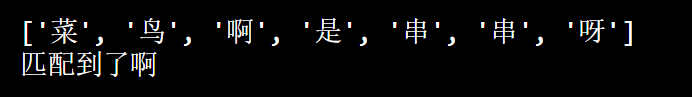匹配任意非数字和字母 返回字符串不包含任何单词字符的匹配项，在每个非单词字符中返回匹配（不在A和Z之间的字符。“！”，“？”空白位等） 例如： import re txt = "菜鸟 是 我嘛？我不信！！" #在每个非单词字符中返回匹配（不在A和Z之间的字符。“！”，“？”空白位等）： x = re.findall("\W", txt) print(x) if x: print("匹配到了!") else: print("匹配不到啊") 运行：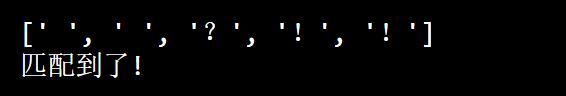匹配结尾 \Z 如果指定的字符位于字符串的末尾，则返回匹配项。 例如： import re txt = "川川是菜鸟啊" x = re.findall("啊\Z", txt) print(x) if x: print("匹配到了哦!") else: print("匹配不到") 集合套装 指定符范围匹配 例如集合：[arn] import re txt = "The rain in Spain" x = re.findall("[arn]", txt) print(x) if x: print("匹配到了!") else: print("匹配不到") 匹配任意范围内小写字母 返回任何小写字符的匹配项，按字母顺序在 a 和 n 之间。 例如: import re txt = "hello wo r l d" x = re.findall("[a-n]", txt) print(x) if x: print("匹配到了!") else: print("匹配不到") 运行：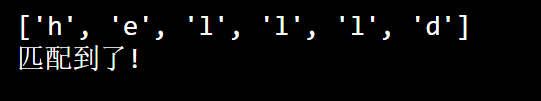同样的道理，依次其它情况如下： [^arn] 返回除 a、r 和 n 之外的任何字符的匹配项  返回存在任何指定数字（0、1、2 或 3）的匹配项 [0-9] 返回 0 到 9 之间任意数字的匹配项 [0-5][0-9] 返回 00 到 59 中任意两位数的匹配项 [a-zA-Z] 按字母顺序返回 a 和 z 之间的任何字符的匹配，小写或大写 [+] 在集合中，+, *, ., |, (),$,{} 没有特殊含义，所以 [+] 的意思是：返回字符串中任意 + 字符的匹配项。这个我i举个例子：

import re

txt = "5+6=11"

#检查字符串是否有任何 + 字符：

x = re.findall("[+]", txt)

print(x)

if x:
print("匹配到了")
else:
print("匹配不到")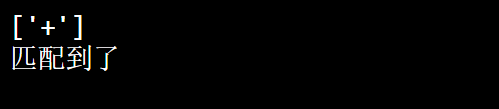匹配对象

import re

#search() 函数返回一个 Match 对象：

txt = "hello world"
x = re.search("wo", txt)
print(x)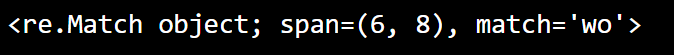Match 对象具有用于检索有关搜索和结果的信息的属性和方法：

span()返回一个包含匹配开始和结束位置的元组。
string返回传递给函数的字符串
group()返回字符串中匹配的部分

span函数

import re

#搜索单词开头的大写“S”字符，并打印其位置

txt = "The rain in Spain"
x = re.search(r"\bS\w+", txt)
print(x.span())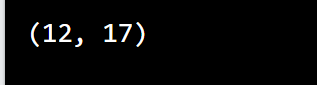string函数

import re

#返回字符串

txt = "The rain in Spain"
x = re.search(r"\bS\w+", txt)
print(x.string)

group函数

import re

#搜索单词开头的大写“w”字符，并打印该单词：

txt = "hello world"
x = re.search(r"\bw\w+", txt)
print(x.group())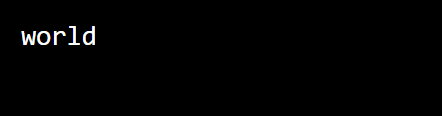08-05
03-29

01-20134
03-221118
11-241018
03-281万+
08-106123
10-014325
10-312639
04-031万+
10-13187
06-074531
04-17169
04-191131
02-21134
02-05199
03-022万+
07-202万+¥2 ¥4 ¥6 ¥10 ¥20余额支付 (余额：-- )扫码支付获取中扫码支付点击重新获取扫码支付1.余额是钱包充值的虚拟货币，按照1:1的比例进行支付金额的抵扣。
2.余额无法直接购买下载，可以购买VIP、C币套餐、付费专栏及课程。余额充值